JMSLTM Numerical Library 7.2.0
com.imsl.stat

Class Covariances

• All Implemented Interfaces:
Serializable, Cloneable

public class Covariances
extends Object
implements Serializable, Cloneable
Computes the sample variance-covariance or correlation matrix.

Class Covariances computes estimates of correlations, covariances, or sums of squares and crossproducts for a data matrix x. Weights and frequencies are allowed but not required.

The means, (corrected) sums of squares, and (corrected) sums of crossproducts are computed using the method of provisional means. Let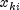denote the mean based on i observations for the k-th variable,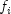denote the frequency of the i-th observation,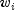denote the weight of the i-th observations, and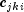denote the sum of crossproducts (or sum of squares if j = k) based on i observations. Then the method of provisional means finds new means and sums of crossproducts as shown in the example below.

The means and crossproducts are initialized as follows: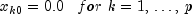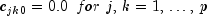where p denotes the number of variables. Letting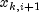denote the k-th variable of observation i + 1, each new observation leads to the following updates forandusing the update constant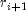: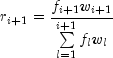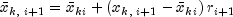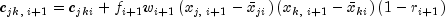The default value for weights and frequencies is 1. Means and variances are computed based on the valid data for each variable or, if required, based on all the valid data for each pair of variables.

Example, Serialized Form
• Field Detail

• CORRECTED_SSCP_MATRIX

public static final int CORRECTED_SSCP_MATRIX
Indicates corrected sums of squares and crossproducts matrix.
Constant Field Values
• CORRELATION_MATRIX

public static final int CORRELATION_MATRIX
Indicates correlation matrix.
Constant Field Values
• STDEV_CORRELATION_MATRIX

public static final int STDEV_CORRELATION_MATRIX
Indicates correlation matrix except for the diagonal elements which are the standard deviations
Constant Field Values
• VARIANCE_COVARIANCE_MATRIX

public static final int VARIANCE_COVARIANCE_MATRIX
Indicates variance-covariance matrix.
Constant Field Values
• Constructor Detail

• Covariances

public Covariances(double[][] x)
Constructor for Covariances.
Parameters:
x - A double matrix containing the data.
Throws:
IllegalArgumentException - is thrown if x.length, and x.length are equal to 0.
• Method Detail

• compute

public final double[][] compute(int matrixType)
throws Covariances.NonnegativeFreqException,
Covariances.NonnegativeWeightException,
com.imsl.stat.Covariances.TooManyObsDeletedException,
com.imsl.stat.Covariances.MoreObsDelThanEnteredException,
com.imsl.stat.Covariances.DiffObsDeletedException
Computes the matrix.
Parameters:
matrixType - An int scalar indicating the type of matrix to compute. Uses class member VARIANCE_COVARIANCE_MATRIX, CORRECTED_SSCP_MATRIX, CORRELATION_MATRIX, STDEV_CORRELATION_MATRIX for matrixType.
Returns:
A double matrix containing computed result.
Throws:
Covariances.NonnegativeFreqException - is thrown if the frequencies are negative.
Covariances.NonnegativeWeightException - is thrown if the weights are negative.
com.imsl.stat.Covariances.TooManyObsDeletedException - is thrown if more observations have been deleted than were originally entered, i.e. the sum of frequencies has become negative.
com.imsl.stat.Covariances.MoreObsDelThanEnteredException - is thrown if more observations are being deleted from "variance-covariance" matrix than were originally entered. The corresponding row,column of the incidence matrix is less than zero.
com.imsl.stat.Covariances.DiffObsDeletedException - is thrown if different observations are being deleted than were originally entered.
• getIncidenceMatrix

public int[][] getIncidenceMatrix()
Returns the incidence matrix. Note that the compute method must be invoked first before invoking this method. Otherwise, the method throws a NullPointerException exception.
Returns:
An int matrix containing the incidence matrix. If method is 0, incidence matrix is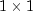and contains the number of valid observations; otherwise, incidence matrix is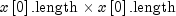and contains the number of pairs of valid observations used in calculating the crossproducts for covariance.
• getMeans

public double[] getMeans()
Returns the means of the variables in x. Note that the compute method must be invoked first before invoking this method. Otherwise, the method throws a NullPointerException exception.
Returns:
A double array containing the means of the variables in x. The components of the array correspond to the columns of x.
• getNumRowMissing

public int getNumRowMissing()
Returns the total number of observations that contain any missing values (Double.NaN). Note that the compute method must be invoked first before invoking this method. Otherwise, the return value is 0.
Returns:
An int scalar containing the total number of observations that contain any missing values (Double.NaN).
• getObservations

public int getObservations()
Returns the sum of the frequencies. Note that the compute method must be invoked first before invoking this method. Otherwise, the return value is 0.
Returns:
An int scalar containing the sum of the frequencies. If missingValueMethod = 0, observations with missing values are not included; otherwise, all observations are included except for observations with missing values for the weight or the frequency.
• getSumOfWeights

public double getSumOfWeights()
Returns the sum of the weights of all observations. Note that the compute method must be invoked first before invoking this method. Otherwise, the return value is 0.
Returns:
A double scalar containing the sum of the weights of all observations. If missingValueMethod = 0, observations with missing values are not included. Otherwise, all observations are included except for observations with missing values for the weight or the frequency.
• setFrequencies

public void setFrequencies(double[] frequencies)
Sets the frequency for each observation.
Parameters:
frequencies - A double array of size x.length containing the frequency for each observation. Default: frequencies[] = 1.
• setMissingValueMethod

public void setMissingValueMethod(int missingValueMethod)
Sets the method used to exclude missing values in x from the computations, where Double.NaN is interpreted as the missing value code.
Parameters:
missingValueMethod - An int scalar indicating the method to use. The methods are as follows:  missingValueMethod Action 0 The exclusion is listwise, default. (The entire row of x is excluded if any of the values of the row is equal to the missing value code.) 1 Raw crossproducts are computed from all valid pairs and means, and variances are computed from all valid data on the individual variables.  Corrected crossproducts, covariances, and correlations are computed using these quantities. 2 Raw crossproducts, means, and variances are computed as in the case of method = 1. However, corrected crossproducts and covariances are computed only from the valid pairs of data.  Correlations are computed using these covariances and the variances from all valid data. 3 Raw crossproducts, means, variances, and covariances are computed as in the case of method = 2. Correlations are computed using these covariances, but the variances used are computed from the valid pairs of data.

• setWeights

public void setWeights(double[] weights)
Sets the weight for each observation.
Parameters:
weights - A double array of size x.length containing the weight for each observation. Default: weights[] = 1.
JMSLTM Numerical Library 7.2.0